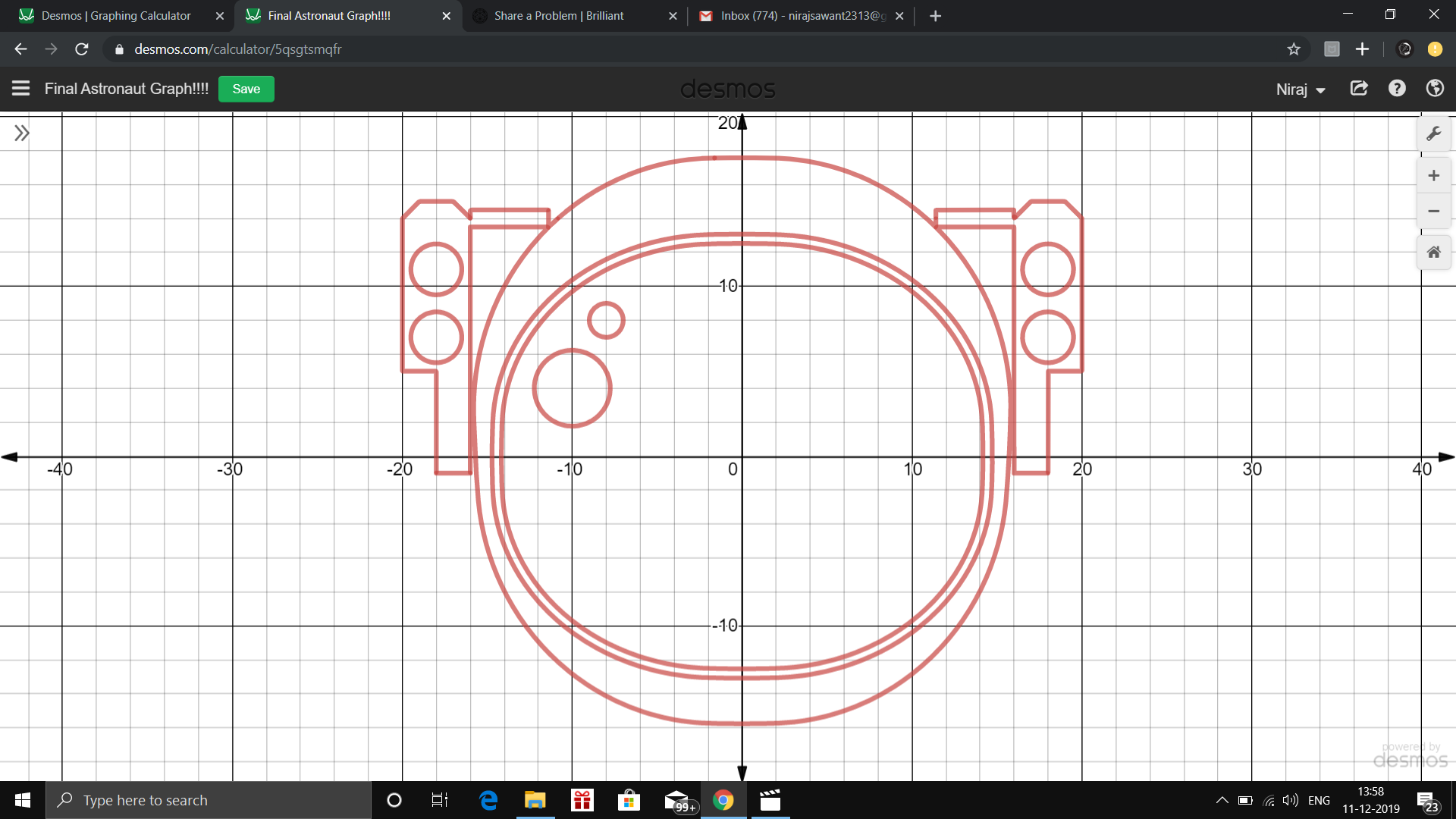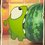# ASTRONAUT Curve

Sorry I posted this as a question.Link to Desmos -$$\url{https://www.desmos.com/calculator/nwyqmc0xlf}$$

THE EQUATION IS BELOW ->

Its confusing to see but like The Batman Curve this is a product of different curves but unlike Batman Curve , you can plot this on DESMOS also!

$\left(\left(\left|x\right|-18\right)^{2}+\left(y-11\right)^{2}-2.25\right)\left(\left(\left|x\right|-18\right)^{2}+\left(y-7\right)^{2}-2.25\right)$

$\left(\left|\dfrac{x+18}{2}+\dfrac{y-9.5}{4.5}\right|+\left|\dfrac{x+18}{2}-\dfrac{y-9.5}{4.5}\right|-2\right)\left(\left|\dfrac{x-18}{2}+\dfrac{y-9.5}{4.5}\right|+\left|\dfrac{x-18}{2}-\dfrac{y-9.5}{4.5}\right|-2\right)$

$\left(\left(\left(\dfrac{\left|x\right|-2+\left|\left|x\right|-2\right|}{23}\right)^{2}+\left(\dfrac{y}{20}\right)^{2}\right)+\left(\left(\dfrac{x}{23}\right)^{2}+\left(\dfrac{\left|y\right|-2+\left|\left|y\right|-2\right|}{20}\right)^{2}\right)-1.5\right)$

$\left(\left(\left(\dfrac{\left|x\right|-2+\left|\left|x\right|-2\right|}{24}\right)^{2}+\left(\dfrac{y}{21}\right)^{2}\right)+\left(\left(\dfrac{x}{24}\right)^{2}+\left(\dfrac{\left|y\right|-2+\left|\left|y\right|-2\right|}{21}\right)^{2}\right)-1.5\right)$

$\left(\left(x+10\right)^{2}+\left(y-4\right)^{2}-5\right)\left(\left(x+8\right)^{2}+\left(y-8\right)^{2}-1\right)\left(\left|\dfrac{x+17}{1}+\dfrac{y-2}{3}\right|+\left|\dfrac{x+17}{1}-\dfrac{y-2}{3}\right|-2\right)$

$\left(\left|\dfrac{x-17}{1}+\dfrac{y-2}{3}\right|+\left|\dfrac{x-17}{1}-\dfrac{y-2}{3}\right|-2\right)$

$\left(\left(\left(\dfrac{\left|x\right|-2+\left|\left|x\right|-2\right|}{26}\right)^{2}+\left(\dfrac{y-4}{27}\right)^{2}\right)+\left(\left(\dfrac{x}{26}\right)^{2}+\left(\dfrac{\left|y\right|-2+\left|\left|y\right|-2\right|-1}{27}\right)^{2}\right)-1.5\right)$

$\left(10^{5}\left(y-14\right)\left(\left(y-14\right)-0.5\left(1-\dfrac{\left|\left|x+18\right|-1\right|}{\left|x+18\right|-1}\right)-0.5\left(\left(x+18\right)\left(\dfrac{\left|x+18\right|}{x+18}\right)-2\right)\left(\dfrac{\left|-\left|x+18\right|+1\right|}{-\left|x+18\right|+1}+\dfrac{\left|\left|x+18\right|-2\right|}{\left|x+18\right|-2}\right)\right)+1\right)$

$\left(10^{5}\left(y-14\right)\left(\left(y-14\right)-0.5\left(1-\dfrac{\left|\left|x-18\right|-1\right|}{\left|x-18\right|-1}\right)-0.5\left(\left(x-18\right)\left(\dfrac{\left|x-18\right|}{x-18}\right)-2\right)\left(\dfrac{\left|-\left|x-18\right|+1\right|}{-\left|x-18\right|+1}+\frac{\left|\left|x-18\right|-2\right|}{\left|x-18\right|-2}\right)\right)+1\right)$

$\left(\left|\dfrac{x+13.7}{2.3}+\dfrac{y-14}{0.5}\right|+\left|\dfrac{x+13.7}{2.3}-\dfrac{y-14}{0.5}\right|-2\right)$

$\left(\left|\dfrac{x-13.7}{2.3}+\dfrac{y-14}{0.5}\right|+\left|\dfrac{x-13.7}{2.3}-\dfrac{y-14}{0.5}\right|-2\right)=0$Note by Niraj Sawant
1 year, 7 months ago

This discussion board is a place to discuss our Daily Challenges and the math and science related to those challenges. Explanations are more than just a solution — they should explain the steps and thinking strategies that you used to obtain the solution. Comments should further the discussion of math and science.

When posting on Brilliant:

• Use the emojis to react to an explanation, whether you're congratulating a job well done , or just really confused .
• Ask specific questions about the challenge or the steps in somebody's explanation. Well-posed questions can add a lot to the discussion, but posting "I don't understand!" doesn't help anyone.
• Try to contribute something new to the discussion, whether it is an extension, generalization or other idea related to the challenge.

MarkdownAppears as
*italics* or _italics_ italics
**bold** or __bold__ bold
- bulleted- list
• bulleted
• list
1. numbered2. list
1. numbered
2. list
Note: you must add a full line of space before and after lists for them to show up correctly
paragraph 1paragraph 2

paragraph 1

paragraph 2

[example link](https://brilliant.org)example link
> This is a quote
This is a quote
    # I indented these lines
# 4 spaces, and now they show
# up as a code block.

print "hello world"
# I indented these lines
# 4 spaces, and now they show
# up as a code block.

print "hello world"
MathAppears as
Remember to wrap math in $$ ... $$ or $ ... $ to ensure proper formatting.
2 \times 3 $2 \times 3$
2^{34} $2^{34}$
a_{i-1} $a_{i-1}$
\frac{2}{3} $\frac{2}{3}$
\sqrt{2} $\sqrt{2}$
\sum_{i=1}^3 $\sum_{i=1}^3$
\sin \theta $\sin \theta$
\boxed{123} $\boxed{123}$

Sort by:

This is niceeeee. How did you come up with such a beastly expression on your own???

- 1 year, 7 months ago

Trial and Error, I basically played around with equations a lot, took me over a month !

- 11 months ago